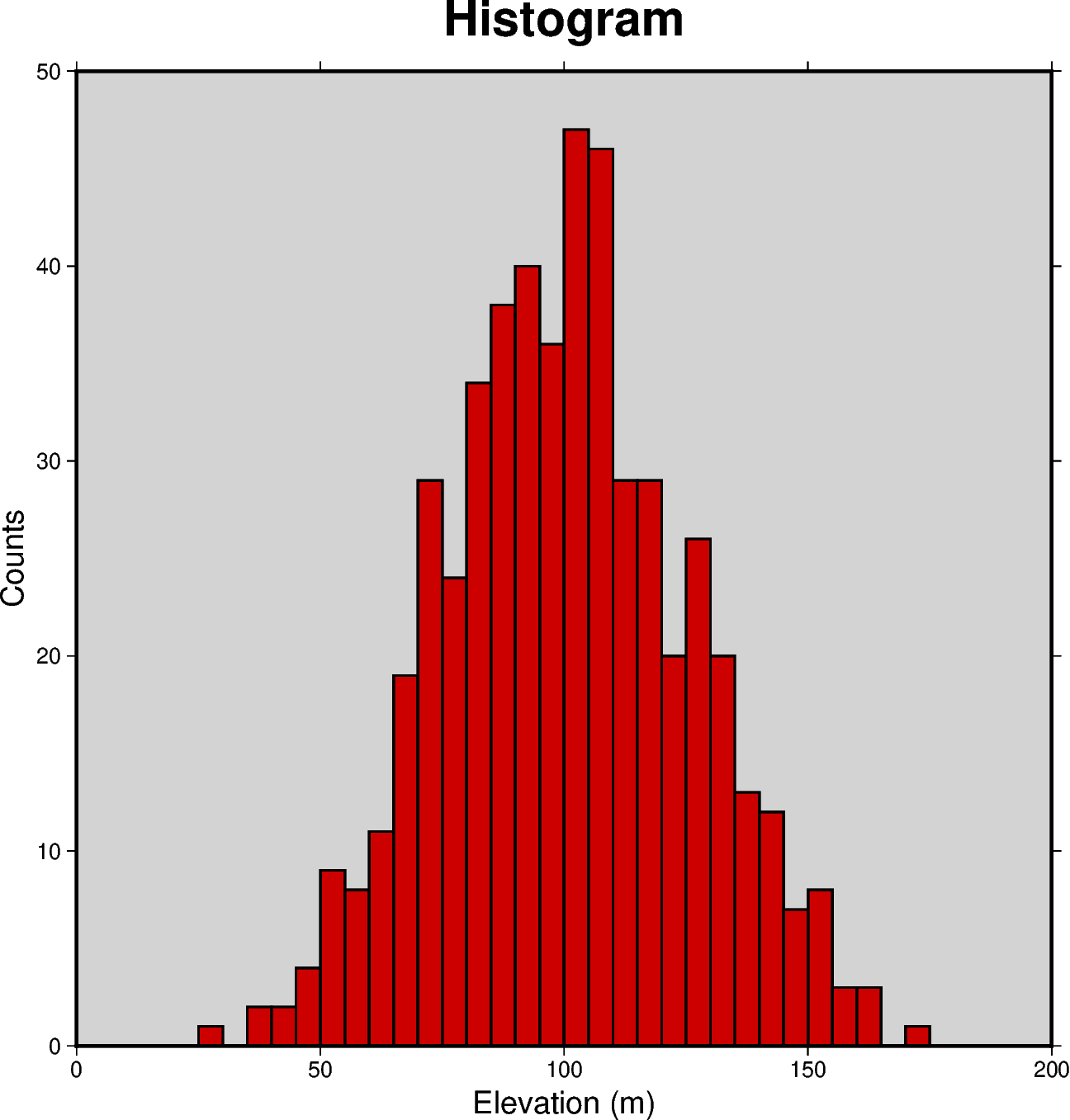# Histogram

The `pygmt.Figure.histogram` method can plot regular histograms. Using the `series` parameter allows to set the interval for the width of each bar. The type of the histogram (frequency count or percentage) can be selected via the `histtype` parameter.```import numpy as np
import pygmt

np.random.seed(100)

# Generate random elevation data from a normal distribution
mean = 100  # mean of distribution
stddev = 25  # standard deviation of distribution
data = mean + stddev * np.random.randn(521)

fig = pygmt.Figure()

fig.histogram(
data=data,
# Define the frame, add a title, and set the background color to
# "lightgray". Add labels to the x-axis and y-axis
frame=["WSne+tHistogram+glightgray", "x+lElevation (m)", "y+lCounts"],
# Generate evenly spaced bins by increments of 5
series=5,
# Use "red3" as color fill for the bars
fill="red3",
# Use the pen parameter to draw the outlines with a width of 1 point
pen="1p",
# Choose histogram type 0, i.e., counts [Default]
histtype=0,
)

fig.show()
```

Total running time of the script: ( 0 minutes 0.545 seconds)

Gallery generated by Sphinx-Gallery